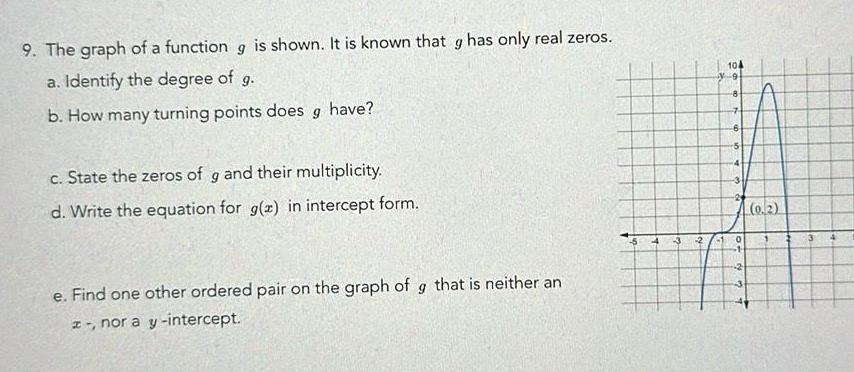Question:

# 9 The graph of a function g is shown It is known that g has

Last updated: 11/17/20239 The graph of a function g is shown It is known that g has only real zeros a Identify the degree of g b How many turning points does g have c State the zeros of g and their multiplicity d Write the equation for g x in intercept form e Find one other ordered pair on the graph of g that is neither an z nor a y intercept 2 41 104 8 7 6 0 0 2